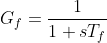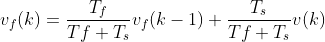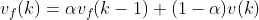# Low-Pass velocity filter theoryv2.0.2

Transfer function of the Low pass filter is controller is:In it discrete form it becomes:where vf(k) is filtered velocity value in moment k, v(k) is the measured velocity in the moment k, Tf is the filter time constant and Ts is the sampling time (or time in between executions of the equation). This low pass filter can be also written in the form:where:This makes it a bit more clear what the time constant `Tf` of the Low pass filter stands for. If your sample time is around 1millisecond (for arduino UNO this can be taken as an average) then setting the `Tf` value to `Tf = 0.01` will result in:

``````alpha = 0.01/(0.01 + 0.001) = 0.91
``````

Which means that your actual velocity measurement v will influence the filtered value vf with the coefficient `1-alpha = 0.09` which is going to smooth the velocity values considerably (maybe even too much, depends of the application).

## Implementation details

Low pass filtering function implemented in the SimpleFOClibrary as a class called `LowPassFilter`. This class receives the time constant in the constructor:

``````LowPassFilter filter = LowPassFilter(0.001); // Tf = 1ms
``````

The filtering function is implemented as follows:

``````// low pass filtering function
float LowPassFilter::operator(float input){
unsigned long timestamp = _micros();
float dt = (timestamp - timestamp_prev)*1e-6f;
// quick fix for strange cases (micros overflow)
if (dt < 0.0f || dt > 0.5f) dt = 1e-3f;

// calculate the filtering
float alpha = Tf/(Tf + dt);
float y = alpha*y_prev + (1.0f - alpha)*x;

// save the variables
y_prev = y;
timestamp_prev = timestamp;
return y;
}
``````

You can use it in code just by calling:

``````float signal_filtered = filter(signal);
``````

And you can change the filtering constant at any time with line:

``````filter.Tf = 0.01; // changed to 10ms
``````

This low pass filter is implemented in the motor class and its time constant can be changed by calling:

``````motor.LPF_velocity.Tf = 0.01;// to set it to 10ms
``````